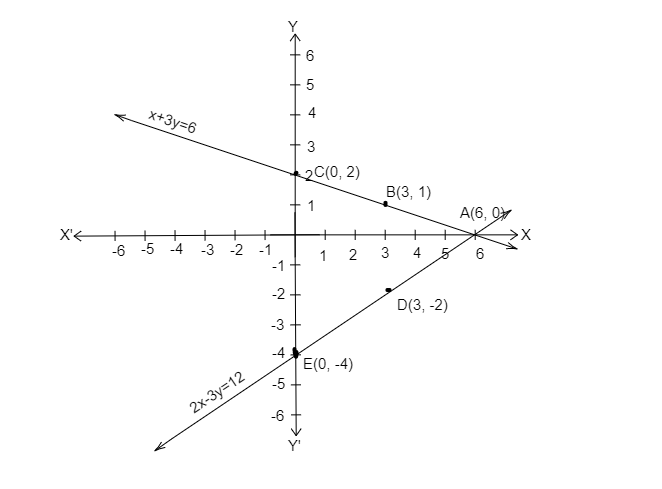# Solve the following pair of linear equations graphically:$x+3y=6;\ 2x-3y=12$

Given: Equations $x+3y=6;\ 2x-3y=12$.

To do: To solve the given equations graphically.

Solution:  given equations are:

$x+3y=6$                 ....................$( 1)$

$2x-3y=12$               ....................$( 2)$

In equation $( 1)$,

$x+3y=6$

$x=6-3y$

Table for equation $( 1)$ for the different value of y.

 x 6 3 0 y 0 1 2

In equation $( 2),$2x-3y=122x=12+3y\frac{12+3y}{2}$Table for equation$( 2)$for the different value of y.  x 0 6 3 y -4 0 -2 by plotting$A( 6,\ 0) ,\ B( 3,\ 1) \ ,\ C( 0,\ 2) ,\ D( 3,\ -2) \ and\ E( 0,\ -4)$on the graph we find both lines intersecting at$ A( 6,\ 0)$.$\therefore \ x=6,\ y=0\$

Updated on: 10-Oct-2022

35 Views